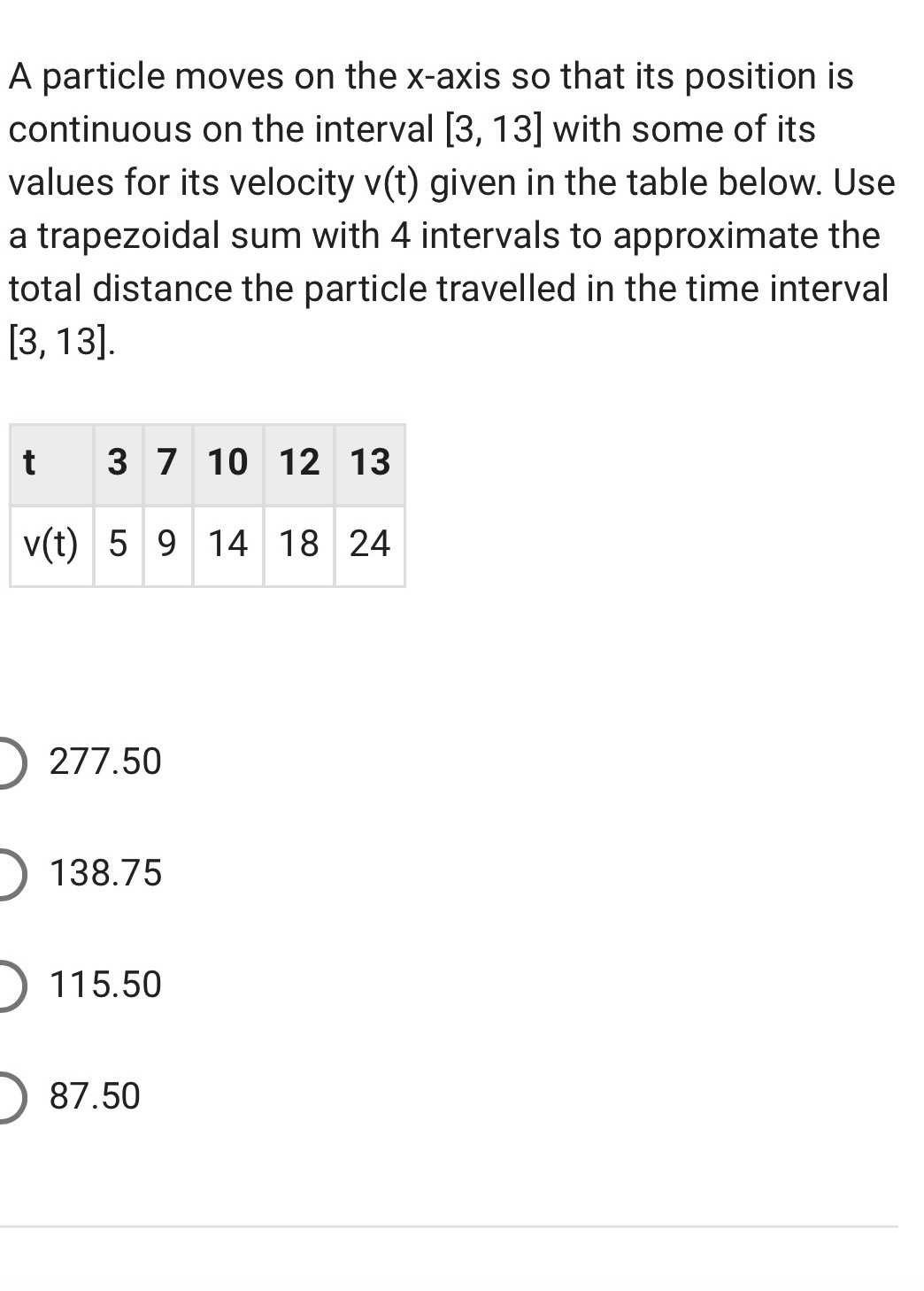### ¿Todavía tienes preguntas de matemáticas?

Pregunte a nuestros tutores expertos
Algebra
PreguntaA particle moves on the $$x$$ -axis so that its position is continuous on the interval $$[ 3,13 ]$$ with some of its values for its velocity $$v ( t )$$ given in the table below. Use a trapezoidal sum with $$4$$ intervals to approximate the total distance the particle travelled in the time interval $$[ 3,13 ]$$ .

$$277.50$$

$$138.75$$

$$115.50$$

$$87.50$$